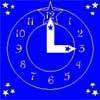#### You may also like### Clock Hands

This investigation explores using different shapes as the hands of the clock. What things occur as the the hands move.### Watch the Clock

During the third hour after midnight the hands on a clock point in the same direction (so one hand is over the top of the other). At what time, to the nearest second, does this happen?### Take the Right Angle

How many times in twelve hours do the hands of a clock form a right angle? Use the interactivity to check your answers.

# How Safe Are You?

##### Age 7 to 11Challenge Level

George and Dominic from St Nicolas C of E Junior School sent us very clearly explained solutions to this problem. Thank you! Here is what they wrote for the first part:

1. For the first question I worked it out by seeing that number 3 was at $180^\circ$ and that meant I needed to divide 180 by 3, giving the angle in between each number. The answer was $60^\circ$. By knowing this I could work out that number 2 was $120^\circ$ away from zero anticlockwise and was $240^\circ$ clockwise from zero.

2. To work this out I did almost the same as I did with question 1 except that I divided 180 by 4 instead. This gave me $45^\circ$ between each number. By multiplying $45^\circ$ by 3 (which is how many numbers 5 is away from zero ) I got $135^\circ$. This meant that I would have to either turn the dial $135^\circ$ clockwise or $225^\circ$ anticlockwise.

3. This was a little easier to work out because number 3 was a quarter of the way round the dial meaning to get to it I either had to turn it $90^\circ$ anticlockwise or $270^\circ$ clockwise.

4. This was harder than the previous questions because there wasn't a number at the $180^\circ$ point. To work it out I divided $360^\circ$ by 9. This gave me how many degrees I would have to turn the dial to get to the next number. The answer was $40^\circ$. This showed me that to get to number 3 I had to turn it either $120^\circ$ anticlockwise or $240^\circ$ clockwise.

5. At first sight this looked really tricky but after thinking about it wasn't. Number 12 is $180^\circ$ away from zero. Half of 12 is 6 and half of 180 is 90, meaning that to get to the number 6 I have to either turn the dial $90^\circ$ anticlockwise or $270^\circ$ clockwise.

George and Dominic continued to explain how they had gone about the second part of the solution:

The next five were the hardest questions because we couldn't see where the zero was at the start.

A. For this question I worked out that 12 was $180^\circ$ from zero and 180 divided by twelve is 15. This means that each section is $15^\circ$ wide. There are 9 spaces between zero and nine which means that to work out the answer we have to times 9 by 15 which equals 135. So to get to 9 I have to turn the dial either $135^\circ$anticlockwise or $225^\circ$ clockwise.

B. We found this one quite easy because again 6 was a quarter of the way round, so this meant that we had to turn the dial $90^\circ$ clockwise or $270^\circ$ anticlockwise.

C. First I worked out that 360 divided by 6 is 60 meaning that each section is $60^\circ$ around the dial. Using this information I worked out that I would have to turn the dial either $120^\circ$ clockwise or $240^\circ$ anticlockwise.

D. On this one I knew that 6 was at $180^\circ$ but you had to work out what 5 was. So I did 180 divided by 6 which equals 30. Then I did 180-30 which equals $150^\circ$ so it was $150^\circ$ anticlockwise and $210^\circ$ clockwise.

E. Firstly for this I divided 360 by 9 which gave me 40. I then multiplied 40 by 5 which gave me 200. Knowing this I could work out that to get to 5 I either have to turn the dial $200^\circ$ anticlockwise or $160^\circ$ clockwise.

We ended the lesson by writing down 3 instructions for someone else to follow which would give our combination. Sophie, another girl in our group, wrote down: Using dial D, turn the dial $240^\circ$ anticlockwise. Then from that point, turn the dial $210^\circ$ clockwise. Then from this point, turn the dial $90^\circ$ anticlockwise. What's my combination?

We worked out that it was 8 1 4.

Very well done to you both. You obviously worked hard on this activity. Thank you too, to Eve and Rachel from Castle Carrock Primary who also sent in well-explained solutions.